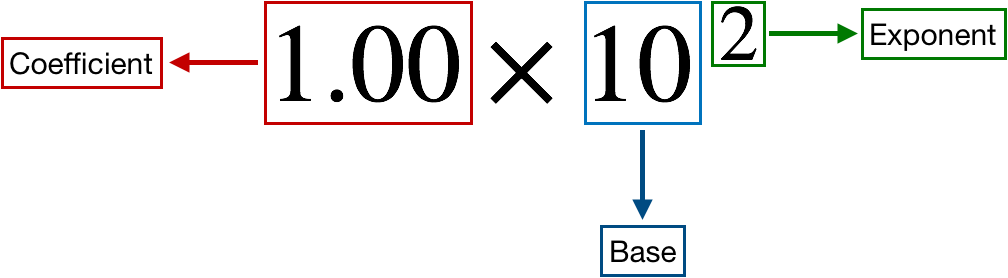# Problem: What is the following number to four significant figures in standard exponential notation: 656,980

###### FREE Expert Solution

We’re being asked to express 656,980 in scientific notation while retaining only 4 significant figures.

Recall that a scientific notation expression is composed of three parts:92% (305 ratings)###### Problem Details

What is the following number to four significant figures in standard exponential notation: 656,980